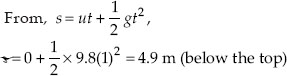Gravitation - NCERT Questions
Q 1.

State the universal law of gravitation.

SOLUTION:

According to universal law of gravitation, every particle in the universe attracts every other particle with a force which is directly proportional to the distance between them. The direction of the force is along the line joining the two particles.

Q 2.

What do you mean by free fall?

SOLUTION:

All objects falling towards earth under the action of gravitational force of earth alone are said to be in free fall.

Q 3.

What do you mean by acceleration due to gravity?

SOLUTION:

The acceleration with which an object fall freely towards the earth is known as acceleration due to gravity. It is denoted by g and its value is 9.8 m s–2.

Q 4.

What are the differences between the mass of an object and its weight?

SOLUTION: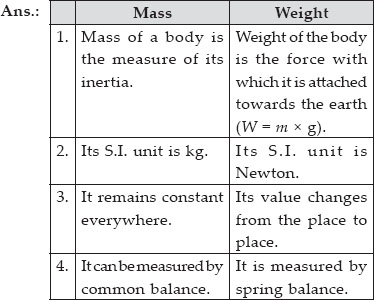Q 5.

Write the formula to find the magnitude of the gravitational force between the earth and an object on the surface of the earth.

SOLUTION:

The formula for the magnitude of gravitational force between the earth and an object on its surface is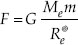where F is the gravitational force.
G is the gravitational constant.
Me is the mass of the earth.
m is the mass of the object on the surface of the earth.
Re is the radius of the earth.

Q 6.

Why is the weight of an object on the moon 1/6th its weight on the earth?

SOLUTION:

The weight of an object depends on the value of acceleration due to gravity g. The value of g on earth is 6 times more than that of moon because, the mass and radius of the earth is more than the mass and radius of the moon.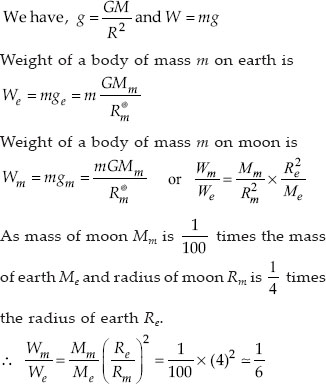Q 7.

How does the force of gravitation between two objects change when the distance between them is reduced to half?

SOLUTION:

Force of gravitation,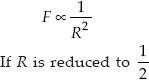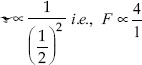The force of gravitation becomes 4 times more.

Q 8.

Gravitational force acts on all objects in proportion to their masses. Why then, a heavy object does not fall faster than a light object?

SOLUTION:

Gravitational force acts on all objects in proportion to their masses. But a heavy object does not fall faster than a light object. This is because of the reason that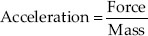or Force = Acceleration × Mass
As force is directly proportional to mass, acceleration is constant for a body of any mass.

Q 9.

What is the magnitude of the gravitational force between the earth and a 1 kg object on its surface? (Mass of the earth is 6 × 1024 kg and radius of the earth is 6.4 × 106 m)

SOLUTION:

The gravitational force between the earth and 1 kg body on its surface is given by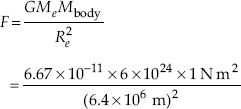This gives, F = 9.8 N

Q 10.

The earth and the moon are attracted to each other by gravitational force. Does the earth attract the moon with a force that is greater or smaller or the same as the force with which the moon attracts the earth? Why?

SOLUTION:

The earth attracts the moon with a force equal to the force with which the moon attracts the earth. This is because as per Newton’s third law of motion, forces of action and reaction are always equal and opposite.

Q 11.

If the moon attracts the earth, why does the earth not move towards the moon?

SOLUTION:

Both the earth and the moon attract each other with the same force. But according to Newton’s second law of motion, acceleration produced in a body by any force is inversely proportional to the mass of the body. Since, mass of the earth is much more than that of the moon, the acceleration produced in the earth is negligible. As a result, it appears as if the earth does not move towards the moon.

Q 12.

What happens to the force between two objects, if
(i) the mass of one object is doubled?
(ii) the distance between the objects is doubled and tripled?
(iii) the masses of both objects are doubled?

SOLUTION:

From the relationship,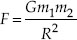(i) If the mass of one object (say body 1) is doubled, then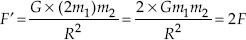Thus, the gravitational force between the two objects gets doubled.
(ii) If the distance between the two objects is doubled, then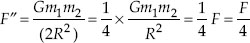Thus, the gravitational force between the two objects becomes one-fourth.
If the distance between the two objects is tripled, then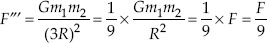Thus, the gravitational force between the two objects becomes one-ninth.
(iii) If the masses of both the objects are doubled, then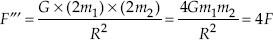Thus, the gravitational force between the two objects becomes 4 times.

Q 13.

What is the importance of universal law of gravitation?

SOLUTION:

Universal law of gravitation is important as it accounts,
(A) for the existence of the solar system, i.e., motion of planets around the sun.
(B) for holding the atmosphere near the surface of the earth.
(C) for the flow of water in rivers.
(D) for rainfall and snowfall.
(e) for occurrence of tides.

Q 14.

What is the acceleration of free fall?

SOLUTION:

All objects moving towards earth on account of gravitational force of earth on them are said to be in free fall. This force produces a uniform acceleration in the object. This is acceleration of free fall and its value is 9.8 m s–2.

Q 15.

What do we call the gravitational force between the earth and an object?

SOLUTION:

The gravitational force between the earth and an object is called the force of gravity or simply earth’s gravity.

Q 16.

mit buys few grams of gold at the poles as per the instruction of one of his friends. He hands over the same when he meets him at the equator. Will the friend agree with the weight of gold bought? If not, why? [Hint : The value of g is greater at the poles than at the equator.]

SOLUTION:

We know that the value of g is greater at the poles than at the equator. So the weight of gold at the equator will be less than the weight of gold at the poles. So it is obvious that the friend at equator will not agree with the weight of gold bought at poles.

Q 17.

Gravitational force on the surface of the moon is only 1/6 as strong as gravitational force on the earth. What is the weight in newtons of a 10 kg object on the moon and on the earth?

SOLUTION:

Here, m = 10 kg
Mass is the same on earth and moon.
Now, weight of the object on earth
We = mge = 10 × 9.8 = 98 N
Weight of the object on moon,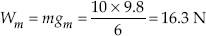Q 18.

A ball is thrown vertically upwards with a velocity of 49 m/s. Calculate
(i) the maximum height to which it rises,
(ii) the total time it takes to return to the surface of the earth.

SOLUTION:

Initial velocity, u = 49 m/s
Acceleration, a = g = – 9.8 m/s2
Velocity at the highest point, v = 0 m/s
(i) If h is the maximum height reached by the ball, then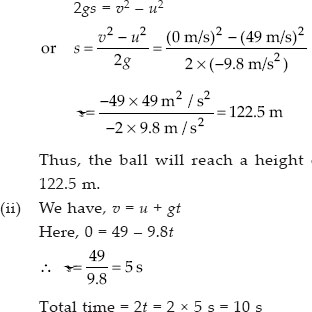Q 19.

A stone is released from the top of a tower of height 19.6 m. Calculate its final velocity just before touching the ground.

SOLUTION:

Initial velocity, u = 0 m s–1
Final velocity, v = ?
Height of the tower, h = 19.6 m
Acceleration due to gravity, g = 9.8 m s–2
Using the equation, v2u2 = 2gs = 2gh
v2 – 0 = 2 × 9.8 m s–2 × 19.6 m
v2 = 19.6 m s–2 × 19.6 m
v2 = (19.6)2 m2 s–2
or v = 19.6 m s–1

Q 20.

A stone is thrown vertically upward with an initial velocity of 40 m/s. Taking g = 10 m/s2,find the maximum height reached by the stone. What is the net displacement and the total distance covered by the stone?

SOLUTION:

Here, u = 40 m/s, g = – 10 m/s2, h = ? v = 0
From v2u2 = 2gh,
0 – (40)2 = 2 (–10)h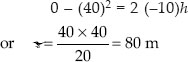As final position of the stone coincides with its initial position, net displacement = 0
Total distance covered by the stone
= h + h = 80 m + 80 m = 160 m

Q 21.

Calculate the force of gravitation between the earth and the sun, given that the mass of the earth = 6 × 1024 kg and of the sun = 2 × 1030 kg. The average distance between the two is 1.5 × 1011 m.

SOLUTION:

Mass of the earth, Me = 6 × 1024 kg
Mass of the sun, Ms = 2 × 1030 kg
Distance between the earth and the sun,
R = 1.5 × 1011 m
Then, the gravitational force between the earth and the sun is given by,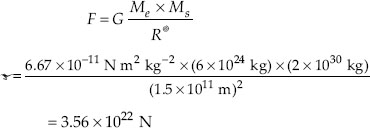Thus, the earth and the sun attract each other by a gravitational force of 3.56 × 1022 N.

Q 22.

A stone is allowed to fall from the top of a tower 100 m high and at the same time another stone is projected vertically upwards from the ground with a velocity of 25 m/s. Calculate when and where the two stones will meet.

SOLUTION:

Here, h = 100 m.
Let the two stones meet after t seconds at a point P which is at a height x above the ground as shown in figure.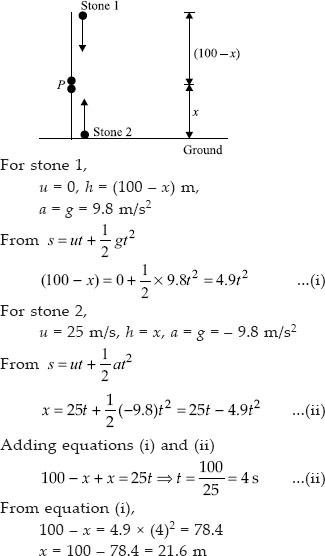Q 23.

A ball thrown up vertically returns to the thrower after 6 s. Find
(A) the velocity with which it was thrown up,
(B) the maximum height it reaches, and
(C) its position after 4 s.

SOLUTION:

Here, time of ascent = time of descent,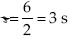(A) u = ?, v = 0, a = – g = – 9.8 m/s2
From v = u + gt,
0 = u – 9.8 × 3 ⇒ u = 29.4 m/s
(B) From v = u + gt,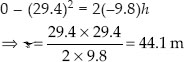(C) t = 3 s, ball is at maximum height.